# pylops.optimization.sparsity.fista#

pylops.optimization.sparsity.fista(Op, y, x0=None, niter=10, SOp=None, eps=0.1, alpha=None, eigsdict=None, tol=1e-10, threshkind='soft', perc=None, decay=None, monitorres=False, show=False, itershow=(10, 10, 10), callback=None)[source]#

Fast Iterative Shrinkage-Thresholding Algorithm (FISTA).

Solve an optimization problem with $$L^p, \; p=0, 0.5, 1$$ regularization, given the operator Op and data y. The operator can be real or complex, and should ideally be either square $$N=M$$ or underdetermined $$N<M$$.

Parameters
Oppylops.LinearOperator

Operator to invert

ynumpy.ndarray

Data

x0: :obj:numpy.ndarray, optional

Initial guess

niterint, optional

Number of iterations

SOppylops.LinearOperator, optional

Regularization operator (use when solving the analysis problem)

epsfloat, optional

Sparsity damping

alphafloat, optional

Step size. To guarantee convergence, ensure $$\alpha \le 1/\lambda_\text{max}$$, where $$\lambda_\text{max}$$ is the largest eigenvalue of $$\mathbf{Op}^H\mathbf{Op}$$. If None, the maximum eigenvalue is estimated and the optimal step size is chosen as $$1/\lambda_\text{max}$$. If provided, the convergence criterion will not be checked internally.

eigsdictdict, optional

Dictionary of parameters to be passed to pylops.LinearOperator.eigs method when computing the maximum eigenvalue

tolfloat, optional

Tolerance. Stop iterations if difference between inverted model at subsequent iterations is smaller than tol

threshkindstr, optional

Kind of thresholding (‘hard’, ‘soft’, ‘half’, ‘soft-percentile’, or ‘half-percentile’ - ‘soft’ used as default)

percfloat, optional

Percentile, as percentage of values to be kept by thresholding (to be provided when thresholding is soft-percentile or half-percentile)

decaynumpy.ndarray, optional

Decay factor to be applied to thresholding during iterations

monitorresbool, optional

Monitor that residual is decreasing

showbool, optional

Display iterations log

itershowtuple, optional

Display set log for the first N1 steps, last N2 steps, and every N3 steps in between where N1, N2, N3 are the three element of the list.

callbackcallable, optional

Function with signature (callback(x)) to call after each iteration where x is the current model vector

Returns
xinvnumpy.ndarray

Inverted model

niterint

Number of effective iterations

costnumpy.ndarray, optional

History of cost function

Raises
NotImplementedError

If threshkind is different from hard, soft, half, soft-percentile, or half-percentile

ValueError

If perc=None when threshkind is soft-percentile or half-percentile

OMP

Orthogonal Matching Pursuit (OMP).

ISTA

Iterative Shrinkage-Thresholding Algorithm (ISTA).

SPGL1

Spectral Projected-Gradient for L1 norm (SPGL1).

SplitBregman

Split Bregman for mixed L2-L1 norms.

Notes

## Examples using pylops.optimization.sparsity.fista#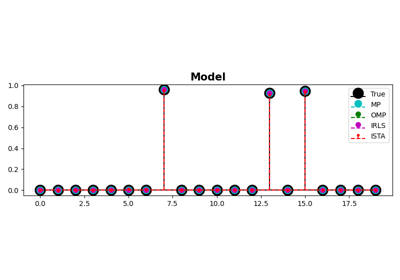MP, OMP, ISTA and FISTA

MP, OMP, ISTA and FISTA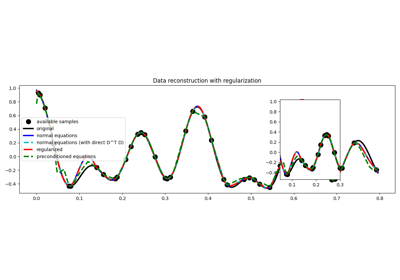03. Solvers

03. Solvers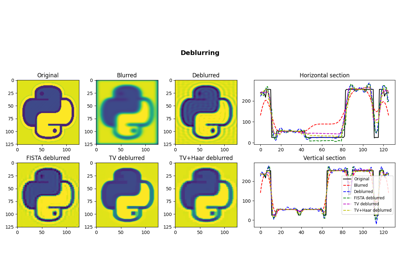05. Image deblurring

05. Image deblurring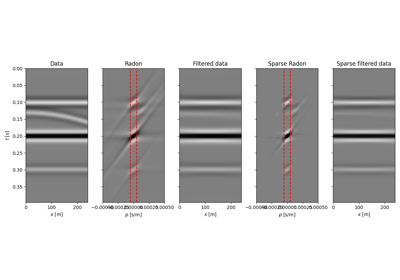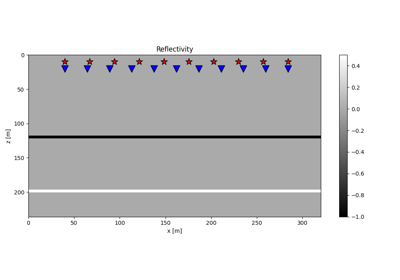15. Least-squares migration

15. Least-squares migration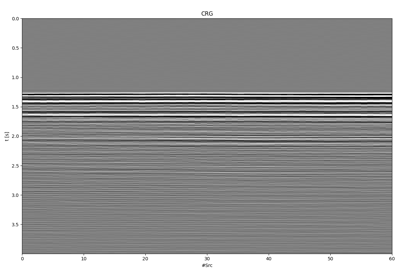18. Deblending

18. Deblending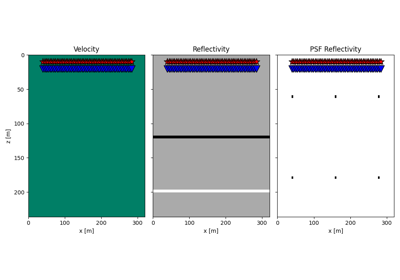20. Image Domain Least-squares migration

20. Image Domain Least-squares migration